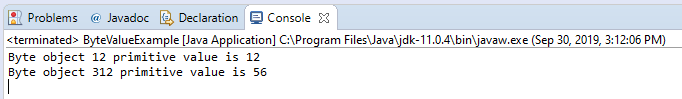## Description

The byteValue() method of Short class Returns the value of this Short as a primitive byte after a narrowing conversion. Take note that short had higher capacity than byte thus a narrowing conversion will take place.

Important Notes:

• The method `byteValue()` overrides byteValue in class Number

## Method Syntax

public short shortValue()

## Method Argument

Data Type Parameter Description
N/A N/A N/A

## Method Returns

The byteValue() method of Short class returns the numeric value represented by this object after conversion to type byte.

## Compatibility

Requires Java 1.1 and up

## Java Short byteValue() Example

Below is a simple java example on the usage of byteValue() method of Short class.

```package com.javatutorialhq.java.examples;

/*
* This example source code demonstrates the use of
* byteValue() method of Short class.
*/

public class ByteValueExample {

public static void main(String[] args) {

// instantiate a new Short object
Short value = 12;

// convert value of Short object type to byte primitive
byte bytePrimitive = value.byteValue();

// print the result
System.out.println("Byte object " + value +
" primitive value is " + bytePrimitive);

// test the narrowing conversion
// byte range is -128 to 127

value = 312;
bytePrimitive = value.byteValue();

System.out.println("Byte object " + value +
" primitive value is " + bytePrimitive);

}

}

```

On the above example we have two result. First is when we created a new Short object with value 12. This is a straightforward conversion as we only converted a Short object into primitive byte. Since 12 is within the range of values allocated for Byte, then it will have the same value.

On the second instance we assign a value of 312 and then we attempt to convert into primitive type. There is no exception encountered but a narrowing conversion has taken place because byte value has only a range of -128 to 127. Since 312 is greater than 127, it will undergo a narrowing conversion.

## Sample Output

Below is the sample output when you run the above example.